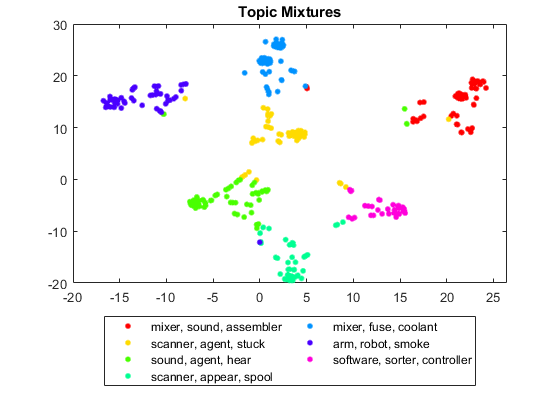# Visualize Document Clusters Using LDA Model

This example shows how to visualize the clustering of documents using a Latent Dirichlet Allocation (LDA) topic model and a t-SNE plot.

A latent Dirichlet allocation (LDA) model is a topic model which discovers underlying topics in a collection of documents and infers word probabilities in topics. The vectors of per-topic word probabilities characterize the topics. You can evaluate document similarity using an LDA model by comparing the per-document topic probabilities, also known as topic mixtures.

Load the LDA model `factoryReportsLDAModel` which is trained using a data set of factory reports detailing different failure events. For an example showing how to fit an LDA model to a collection of text data, see Analyze Text Data Using Topic Models.

```load factoryReportsLDAModel mdl```
```mdl = ldaModel with properties: NumTopics: 7 WordConcentration: 1 TopicConcentration: 0.5755 CorpusTopicProbabilities: [0.1587 0.1573 0.1551 0.1534 0.1340 0.1322 0.1093] DocumentTopicProbabilities: [480×7 double] TopicWordProbabilities: [158×7 double] Vocabulary: [1×158 string] TopicOrder: 'initial-fit-probability' FitInfo: [1×1 struct] ```

Visualize the topics using word clouds.

```numTopics = mdl.NumTopics; figure tiledlayout("flow") title("LDA Topics") for i = 1:numTopics nexttile wordcloud(mdl,i); title("Topic " + i) end```### Visualize Document Clusters Using t-SNE

The t-distributed stochastic neighbor embedding (t-SNE) algorithm projects high-dimensional vectors to 2-D space. This embedding makes it easy to visualize similarity between high-dimensional vectors. By plotting the document topic mixtures according to the t-SNE algorithm, you can visualize the clustering of similar documents.

Project the topic mixtures in the `DocumentTopicProbabilties` property into 2-D space using the `tsne` function.

`XY = tsne(mdl.DocumentTopicProbabilities);`

For the plot groups, identify the top topic for each document.

`[~,topTopics] = max(mdl.DocumentTopicProbabilities,[],2);`

For the plot labels, find the top three words for each topic.

```for i = 1:numTopics top = topkwords(mdl,3,i); topWords(i) = join(top.Word,", "); end```

Plot the projected topic mixtures using the `gscatter` function. Specify the top topics as the grouping variable and display a legend with the top words for each topic.

```figure gscatter(XY(:,1),XY(:,2),topTopics) title("Topic Mixtures") legend(topWords, ... Location="southoutside", ... NumColumns=2)```The t-SNE plot highlights clusters occurring in the original high-dimensional data.